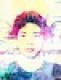## 函數（`Function`）

VBA 的函數（`Function`）跟子程序（`Sub`）類似，比較不一樣的地方是函數在執行完之後會有一個傳回值，而子程序則沒有，以下是一個簡單的函數範例：

```' 自行定義的函數
Function myFun(x As Integer, y As Integer) As Integer
myFun = x + y
End Function```

```Sub Hello()
Dim a As Integer
a = myFun(3, 4)
MsgBox a
End Sub```

### Excel 使用 VBA 函數

VBA 的函數定義好之後，除了可以在一般的 VBA 程式碼中呼叫之外，也可以直接在 Excel 中使用，其使用方式就跟一般的 Excel 函數一樣，在儲存格中輸入等於，再加上自訂的函數名稱以及輸入的資料：

### 預設參數值

```' 自行定義的函數
Function myFun2(Optional x As Integer = 3, Optional y As Integer = 4) As Integer
myFun = x + y
End Function```

```' 呼叫自行定義的函數
Sub Hello()
Dim a As Integer

' 計算 3 + 4
a = myFun2()
MsgBox a

' 計算 20 + 4
a = myFun2(20)
MsgBox a

' 計算 20 + 40
a = myFun2(20, 40)
MsgBox a
End Sub```

### 傳值與傳參考呼叫

VBA 函數的傳值與傳參考呼叫原則與子程序相同，以下是函數的傳值與傳參考呼叫範例：

```' 傳參考函數
Function myFun3(ByRef x As Integer) As Integer
x = x + 1
myFun3 = x
End Function

' 傳值函數
Function myFun4(ByVal x As Integer) As Integer
x = x + 1
myFun4 = x
End Function```

```Sub Hello()
Dim a As Integer, b As Integer

a = 5
b = myFun3(a)
MsgBox a

a = 5
b = myFun4(a)
MsgBox a

End Sub```

## 應用範例

### 偶數加總

```Function SumEvenNumbers(r As Range) As Integer
Dim c As Range
SumEvenNumbers = 0
' 將範圍內的每一個儲存格資料取出
For Each c In r
' 檢查是否為偶數
If c.Value Mod 2 = 0 Then
' 將偶數加總起來
SumEvenNumbers = SumEvenNumbers + c.Value
End If
Next c
End Function```

### 8 留言

1.#### Caspar

感謝您的用心提供VBA教學，對我很有幫助!

2.#### Amity

感受到您的用心，在您的部落格裏學到不少東西。謝謝!

3.#### Sum

你好, 我想在做一個macro,
1)在Table-A, 選取C2的文字/數字,
2)然後在Table-B上搜尋和Table-A,C2相同的字,
3)然後在Table-B的行數(row)(和C2文字相同的行數)
,最後一個沒有資料的欄位(column)的儲存格,
4)將Table-A, A2的數字, copy寫在Table-B, 最後一個沒有資料的欄位(column)的儲存格.

煩請大大幫忙以下可以怎樣改, 謝謝.
以下”Cells.Find(What:=”BB”, _”,這個”BB”是每次都不同的,

Sub Macro1()
Application.ScreenUpdating = False

Range(“C2”).Select
Selection.Copy
Windows(“Table A.xlsx”).Activate
Cells.Find(What:=”BB”, _
After:=ActiveCell, _
LookIn:=xlFormulas, _
LookAt:=xlPart, _
SearchOrder:=xlByRows, _
SearchDirection:=xlNext, _
MatchCase:=False, _
MatchByte:=False, _
SearchFormat:=False).Activate

Application.CutCopyMode = False
Windows(“Table B.xlsm”).Activate
Range(“A2”).Select
Selection.Copy
Windows(“Table A.xlsx”).Activate
‘Range(“D19”).Select
‘ActiveSheet.Paste
‘Application.CutCopyMode = False
‘Windows(“Table B.xlsm”).Activate
Application.ScreenUpdating = True
End Sub

4.#### Stanley

5.#### CCLIU

非常實用得網站，謝謝您

6.#### Patrick

感受到您的用心，在您的部落格裏增長不少實力，很感謝您!

7.#### youshan

您好，朋友推薦我學 VBA，找到您的網站，覺得非常幸運，感謝您：）
想請問：
Sub hello()
‘ 計算 3 + 4
mySub2

‘ 計算 1 + 4
mySub2 1

‘ 計算 1 + 2
mySub2 1, 2
End Sub

請問如果要「計算 3+2」 呢？我的認知是「mySub2 1」的意思是把「3,4」中的第一個替換為「1」，但如果我只想替換掉「4」，可是想保留「2」呢？謝謝您！

8.#### youshan

您好，

朋友推薦我學 VBA，發現您的網站寫的非常簡明易懂，感謝您！

想請問：

Sub hello()
‘ 計算 3 + 4
mySub2

‘ 計算 1 + 4
mySub2 1

‘ 計算 1 + 2
mySub2 1, 2
End Sub

請問如果我是想要「3+7」呢？也就是，我想替換掉「3,4」中的第二個數字，但保留第一個數字 “3”。謝謝您！